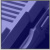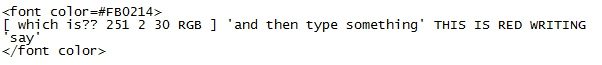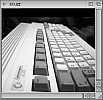ScuzzBlog: Diaries August 2019

Entry 15th August 2019: Post 01: No thumb needed with hexadecimal.

Hi

Brushing up on my hexadecimal tonight and struggling as ever with
counting the letters on my finger. Then read the actual Wiki for the
system and there was a great image of a hand with extended four fingers
and made use of the creases in our fingers. So excluding the thumbs it
goes 0 = tip of first finger and 3 creases takes you to 3. Then next 4
to 7 and then 8,9 and then A and B. With the last finger C,D,E,F. With
F representing 15 obviously.

Now to the maths.....

eg Convert 673 to Hex

So 673 divided by 16 = 42.0625 so times .0626x16=1 and 1 is our first
remainder. Now divide 42 by 16 = 2.625 and so times .625x16=10 and ten
is [ uses finger ] A as our second remainder.

Next divide 2 by 16 which goes by 0 and remainder .125 x16 = 2 and that
is the last remainder.

The hex number is the remainder numbers in Hex so 10 is A [ extends
fingers , third finger second crease ]

Take the least significant number at the base and the Hex is 2A1 or the
remainder numbers in Hex.

OK reverse engineer ..2A1

1 is 1 =1 the first number is the ones
A or 10x16=160 the second number is the twos so number x16
2 x 16x16=512 the third is the thirds so number x 16x16
So adding up 1 + 160 + 512 = 673

And finally the highest Hex number in two symbols is FF or 255
255/16=15.9375 [ .9375x16=15 ] extends fingers and its the last on the
last finger or F as the remainder.

Now take 15 and divide by 16 which is 0 and remainder 0.9375. Times by
16 =15. Finger time and F again.
So the number is FF.

If it was 1 number higher ie 256 then the Hex would be 100 and so when
we specify colours in web pages we use the three max hex numbers of
say FFFFFF which is white or 000000 which is black.

FFFFFF or increments of 255 of red green and blue RGB 255,255,255 or
White FF,FF,FF and all colours at max value gives white. Whereas take
the least value of all colours ie 000000 and we get black.

Just open a Notepad or text editor and type the words?? ...THIS IS RED WRITING

.. and then save simply as say TEST.HTM and then fire up in a browser
and you should see red writing.

Now all I gotta do is find a use for my thumbs.

I did start to get into binary but that got me total confused. All
started cus I watching a video of the inner workings of a microchip.

I can now put my fingers away.

the use of a computer in the early days required a good understanding
of maths. To get the computer to do anything you needed to be able
to program using mathematical formula and know how to target and
manipulate data. And yet today the art of actual computing is almost
zero in the context of modern computers, tablets and mobile phones.
The need to actually undertake any mathematical evaluation to execute
a program is like unnecessary for your average home pc user.

Pick up any copy of Input from the day and you will not find a page
that isn't laden with hundreds and hundreds of numbers. The use of
hexadecimal  was just a way of reducing the number of those characters
and numbers needed to reflect variables.

I first got fascinated with the hex concept with a program I had
on the PCW9512 called Toolbox which involved me using a hex dump
to modify, correct and revert coding to erase or un-erase files.
There was no simple way to use a recycle bin and therefore going
into the code for the disk is all you could do. But in looking at
the code I really did start to visualise the disk structure in a
whole different way. That is why I loved CP/M cus it really was a
disk manipulation tool at its finest. Much better than DOS.

But like I say it's all died over the years. No longer required to
it seems, though programmers probably still dabble.

One final comment on the magic numbers. There are some numbers that
just leave a very heavy imprint on the brain. And for me there can
be none more potent than this string of values ...

1,2,4,8,16,32,64,128,256 and 512.

From computer SIMMs to actual computer names for a long time they
represented the steady growth of your 16K machine on to the C64 and
C128 etc. Magic numbers.

So computer life was always 16 to the something.If you can only see this CONTENT window
then click the image above for the full site

Last updated 15th August 2019

Chandraise KingdomKeep the Faith
scuzzscink 2019